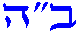Home The Visual Torah Codes The Structural Formulas of Creation# Structures of formulas of the Torah

Structural formulas identify the location of bonds between components of system. Structures of formulas, encoded in the Torah, are geometric representations of components of Torah codes. A full filling of the letter consist of components, component consist of elements. Structural formula of full filling of the letter consist of components and bonds between components.

## Seven types of structures of the Torah codes

There are seven types of structures of the Torah codes: one-dimensional structures of formulas of letters, one-dimensional structures of formulas of a word, one-dimensional structures of formulas of words, two-dimensional structures of formulas of a word, two-dimensional structures of formulas of words, three-dimensional structures of formulas of words, combined one-two or two-three dimensional structures of formulas of word/words.
1. One-dimensional structural formulas of letters. One-dimensional structures are linear structures of letters.
2. One - dimensional structural formulas of word/words. Linear structural formulas of word/words are formed by bonding structural formulas of their letters.
3. Two-dimensional structural formulas of word/words. Two-dimensional structural formulas has two types of bonds: linear bonds between components of structural formulas of letters and external bonds between components of structural formulas of neighboring letters. External bonds can be of four types, based on : similarity conformity, a simple conformity, a one-to-one conformity, a full conformity.
4. Three-dimensional structural formulas of words. Three-dimensional structural formulas has three type bonds: linear bonds between components of structural formulas of letter, external bonds between components of structural formulas of neighboring letters of word, external bonds between components of structural formulas of respective letters of words. External bonds of neighboring letters and respective letters can be of four types: similarity conformity, simple conformity, one-to-one conformity, full conformity. Three-dimensional structural formulas is like a three-dimensional lattice. Frontal planes corresponds to two-dimensional structural formulas of words. All planes from upper to lower correspond to two-dimensional structures of respective letters, with one letter from each word.
5. Combined one-two dimensional structural formulas of word and combined one-two or two-three dimensional structural formulas of words. Combined one-two dimensional structural formulas of word are two - dimensional structure consist of one - dimensional structural formula for a few first letters of word and two dimensional structural formula for remaining letters of word.
And also, such two - dimensional structure can be consist of a few one - dimensional structural formulas of word or a few one - dimensional structural formulas of word and two dimensional structural formula of word. Combined one-two dimensional structural formulas of words are two dimensional structures that have two type bonds: linear bonds between components of structural formulas of letters and external bonds between components of structural formulas of neighboring letters. External bonds of neighboring letters and respective letters can be of four types: similarity conformity, simple conformity, one-to-one conformity, full conformity . In combined one-two dimensional structures, the words are formed by two-dimensional structures, where external bonds of neighboring letters and linear structural formulas of respective letters, are connected together.## Structural formulas of creation is platform for investigation

Structural formulas of creation bears in it a powerful potential to transform the world we live in. Structural formulas of creation is the world's most power platform for investigation in science. Our mission is devoted to the future wherein, thanks to the authentic knowledge of the Jewish Wisdom, the world community will achieve prosperity and secure existence. Want to contribute to the structures formulas of creation? Suggest any conjectures, concerning about it! Join us ! Come work for the world's most perspective research's platform and do a new discovery.Thank you for supporting our work.

## The book "Structural formulas of creation"

The book "Structural formulas of creation" shows that there is more to the Torah than meets the eye, namely, structural formulas of the creation (Torah codes) which are like formulas of chemical bonds between atoms, ions or molecules. The Torah is realistically capable of offering numerous discoveries and innovations of utmost importance for the future. This book contains 452 structural formulas of creation.
Cost: \$34.50 USD , Free International Shipping.Book Review

### Suggestions

 Privacy SiteMap